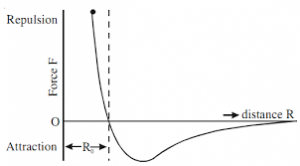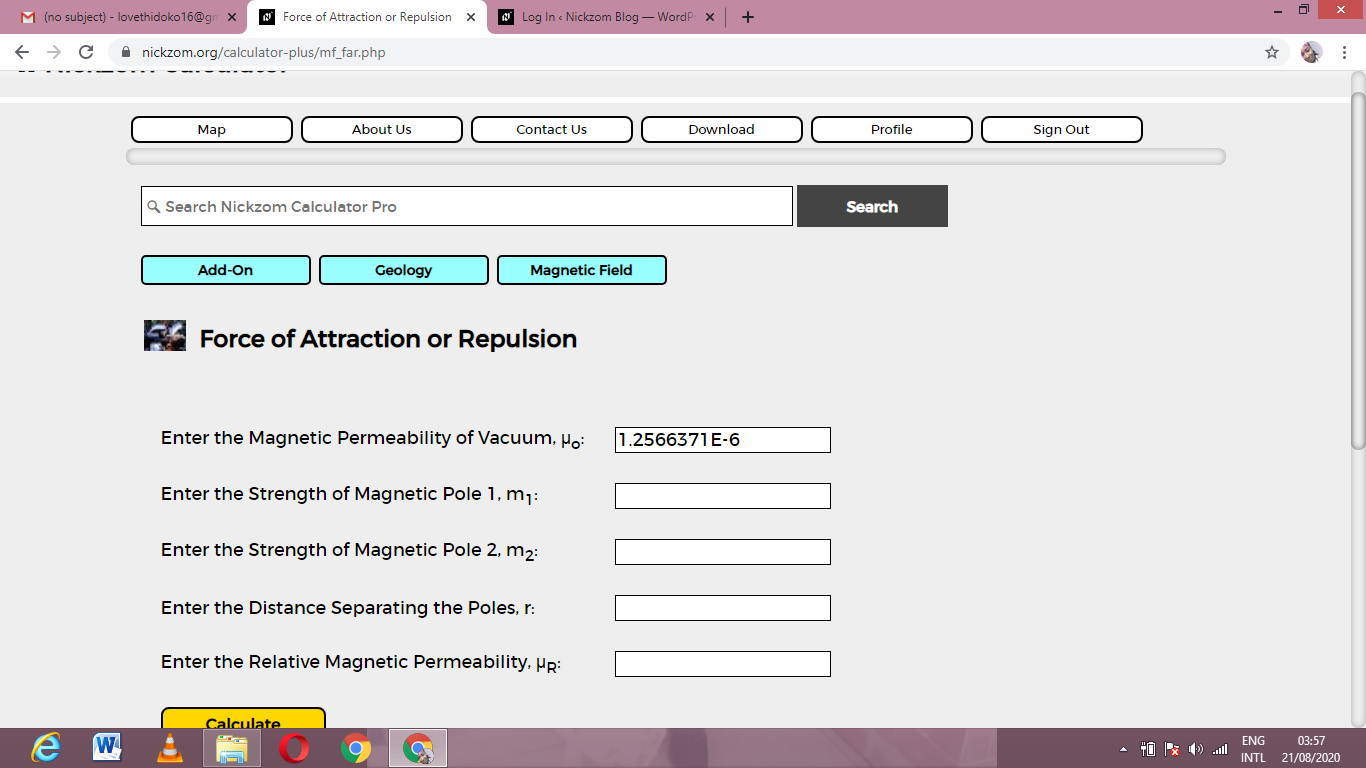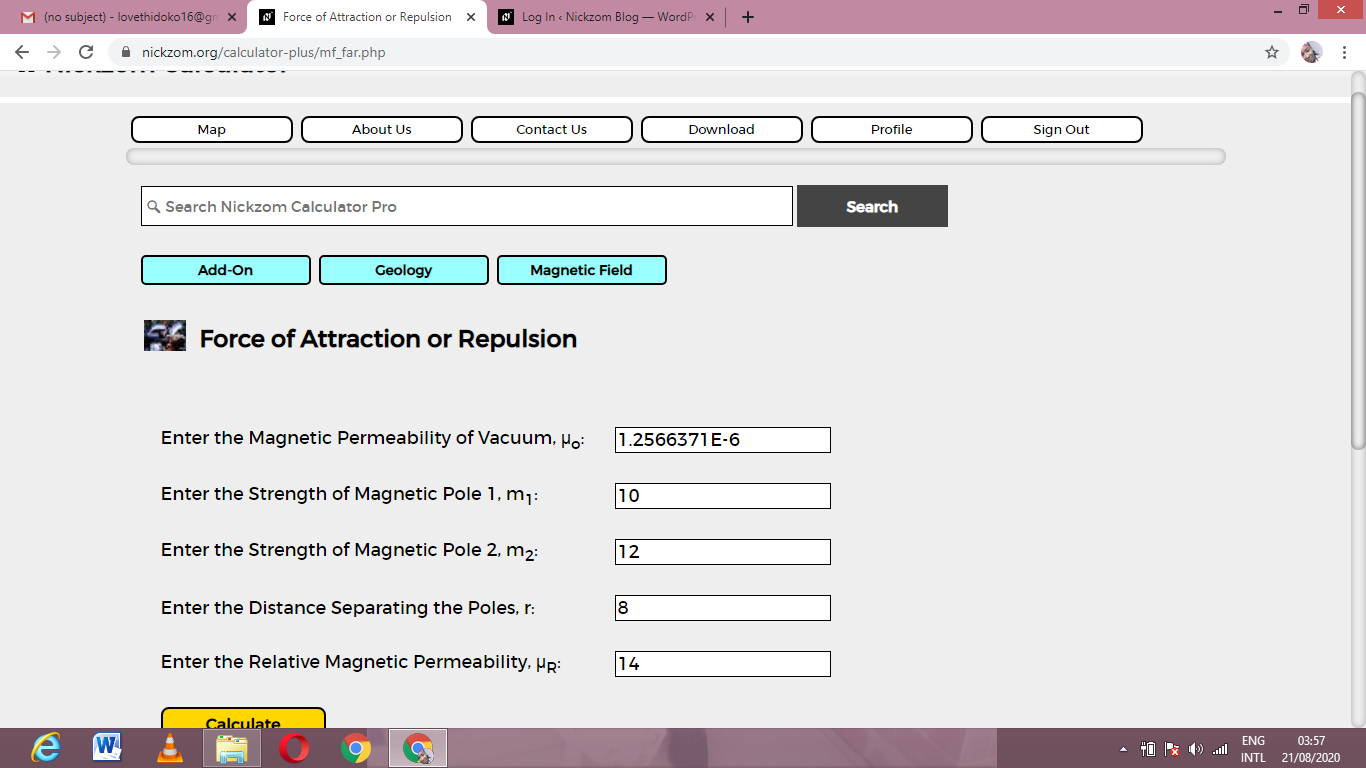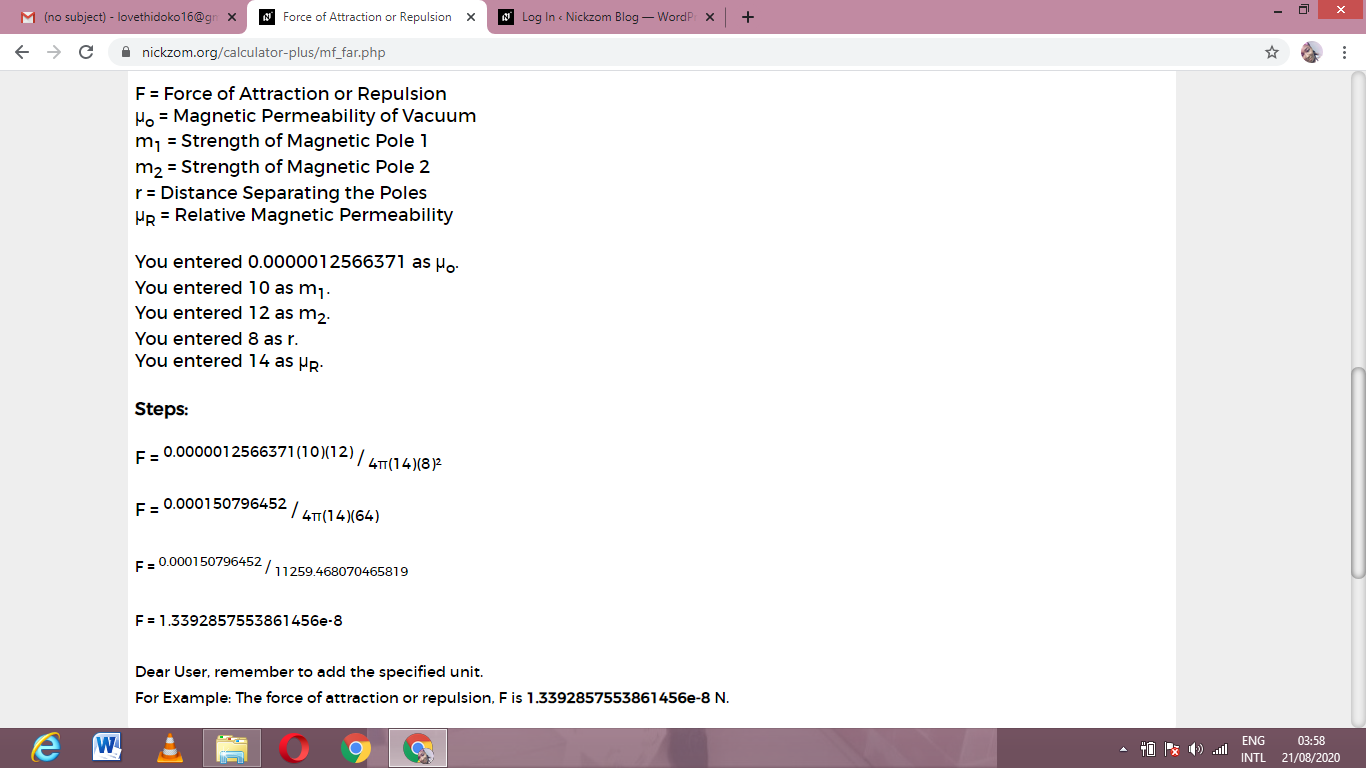# How to Calculate and Solve for Force of Attraction or Repulsion | Magnetic FieldThe image above represents force of attraction or repulsion.

To compute for force of attraction or repulsion, five essential parameters are needed and these parameters are Magnetic Permeability of Vacuum (μo), Strength of Magnetic Pole 1 (m1), Strength of Magnetic Pole 2 (m2), Distance Seperating the Poles (r) and Relative Magnetic Permeability (μR).

The formula for calculating force of attraction or repulsion:

F = μom1m2 / 4πμRr²

Where:

F = Force of Attraction or Repulsion
μo = Magnetic Permeability of Vacuum
m1 = Strength of Magnetic Pole 1
m2 = Strength of Magnetic Pole 2
r = Distance Separating the Poles
μR = Relative Magnetic Permeability

Let’s solve an example;
Find the force of attraction or repulsion when the magnetic permeability of vaccum is 1.2566371E-6, strength of magnetic pole 1 is 10, strength of magnetic pole 2 is 12, distance seperating the poles is 8 and the relative magnetic permeability is 14.

This implies that;

μo = Magnetic Permeability of Vacuum = 1.2566371E-6
m1 = Strength of Magnetic Pole 1 = 10
m2 = Strength of Magnetic Pole 2 = 12
r = Distance Separating the Poles = 8
μR = Relative Magnetic Permeability = 14

F = μom1m2 / 4πμRr²
F = 0.0000012566371(10)(12) / 4π(14)(8)²
F = 0.000150796452 / 4π(14)(64)
F = 0.000150796452 / 11259.468
F = 1.339e-8

Therefore, the force of attraction or repulsion is 1.339e-8.

Nickzom Calculator – The Calculator Encyclopedia is capable of calculating the force of attraction or repulsion.

To get the answer and workings of the force of attraction or repulsion using the Nickzom Calculator – The Calculator Encyclopedia. First, you need to obtain the app.

You can get this app via any of these means:

To get access to the professional version via web, you need to register and subscribe for NGN 2,000 per annum to have utter access to all functionalities.
You can also try the demo version via https://www.nickzom.org/calculator

Apple (Paid) – https://itunes.apple.com/us/app/nickzom-calculator/id1331162702?mt=8
Once, you have obtained the calculator encyclopedia app, proceed to the Calculator Map, then click on Geology under Add-on.Now, Click on Magnetic Field under GeologyNow, Click on Force of Attraction or Repulsion under Magnetic FieldThe screenshot below displays the page or activity to enter your values, to get the answer for the force of attraction or repulsion according to the respective parameters which are the Magnetic Permeability of Vacuum (μo), Strength of Magnetic Pole 1 (m1), Strength of Magnetic Pole 2 (m2), Distance Seperating the Poles (r) and Relative Magnetic Permeability (μR).Now, enter the values appropriately and accordingly for the parameters as required by the Magnetic Permeability of Vacuum (μo) is 1.2566371E-6, Strength of Magnetic Pole 1 (m1) is 10, Strength of Magnetic Pole 2 (m2) is 12, Distance Seperating the Poles (r) is 8 and Relative Magnetic Permeability (μR) is 14.Finally, Click on CalculateAs you can see from the screenshot above, Nickzom Calculator– The Calculator Encyclopedia solves for the force of attraction or repulsion and presents the formula, workings and steps too.